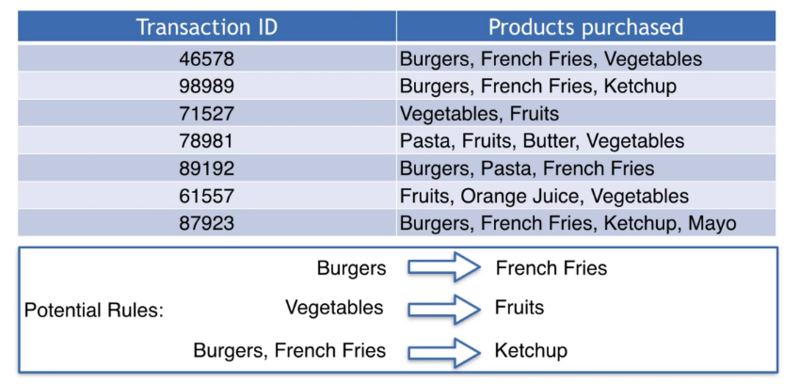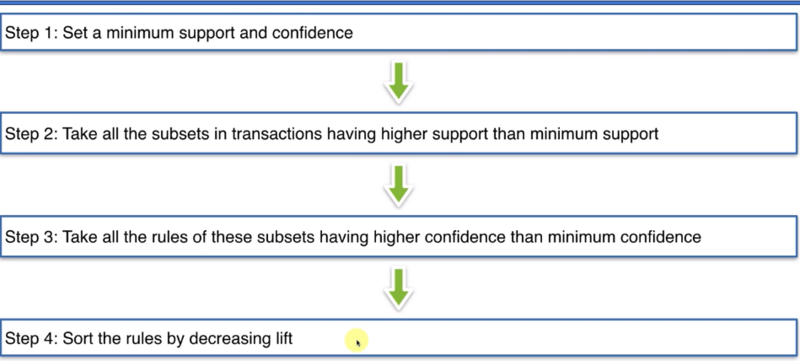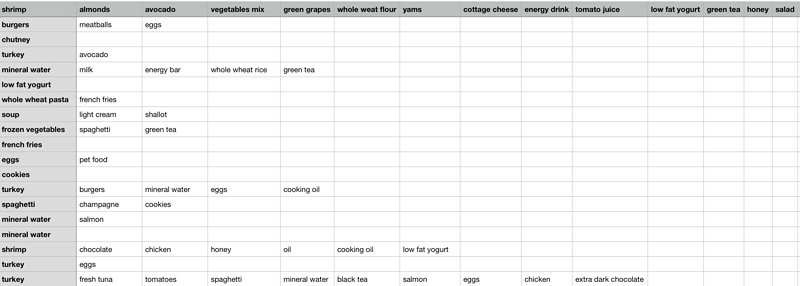## 概念

lift(I_1 -> I_2) = \frac{confidence(I_1->I_2)}{support(I_2)}

1. 设置一个最低的support和confidence
2. 选择所有support比刚刚设置的要大的商品
3. 根据刚刚已经选择的商品，选择所有比刚刚定义的最小confidence要高的所有规则的集合
4. 把刚刚的规则从大到小排序，选出提升度最高的几个。## 代码实现from apyori import apriori
import pandas as pd

transactions = []
for i in range(0, 7501):
transactions.append([str(dataset.values[i, j]) for j in range(0, 20)])

# Training Apriori on the dataset

rules = apriori(transactions, min_support=0.003, min_confidence=0.2, min_lift=3, min_length=2)

# Visualising the results
results = list(rules)
myResults = [list(x) for x in results]